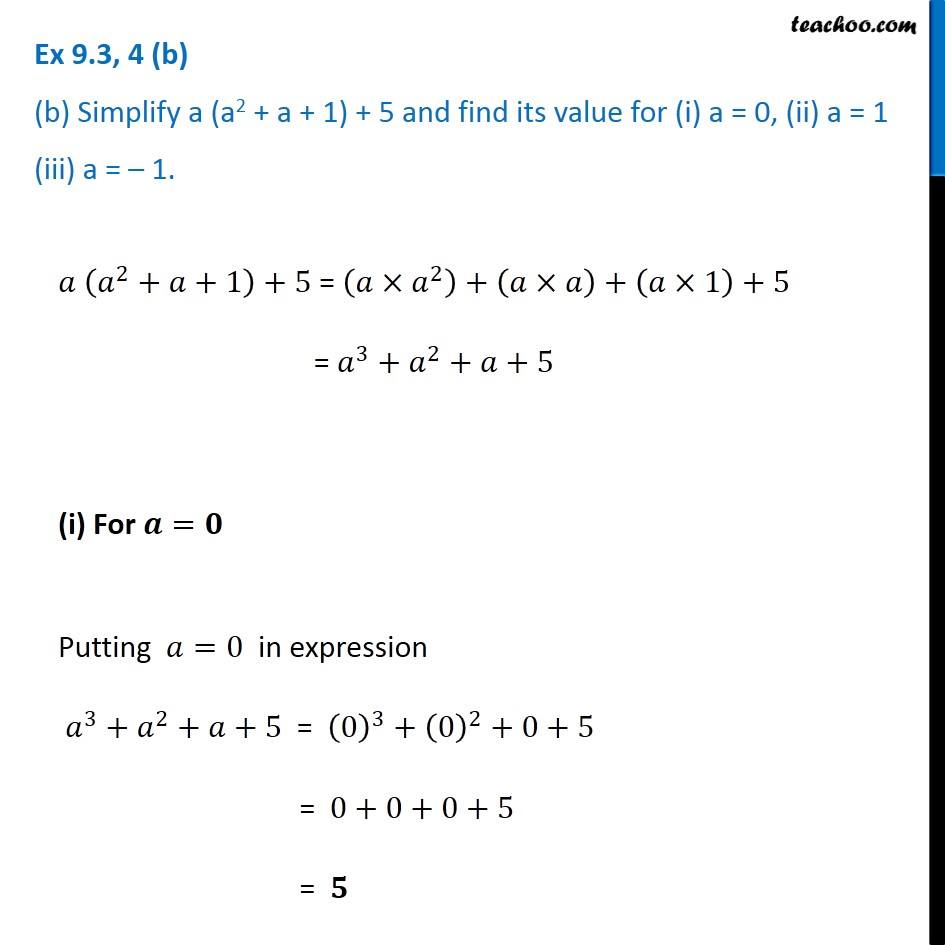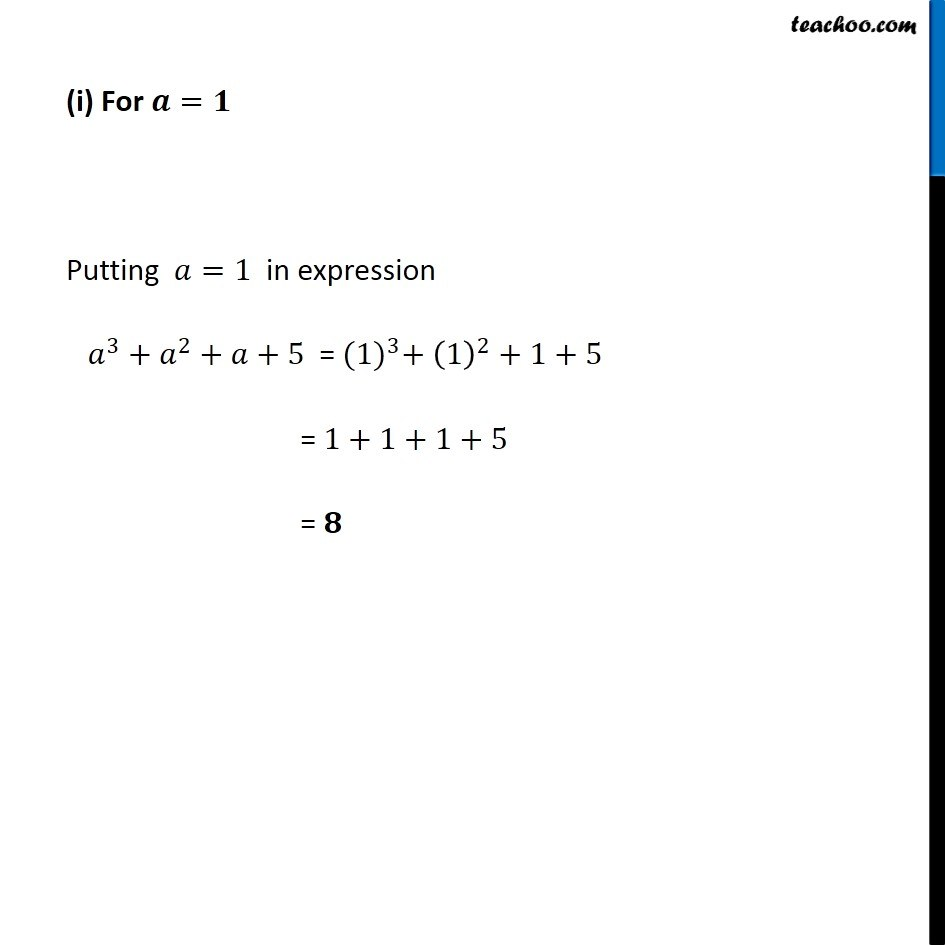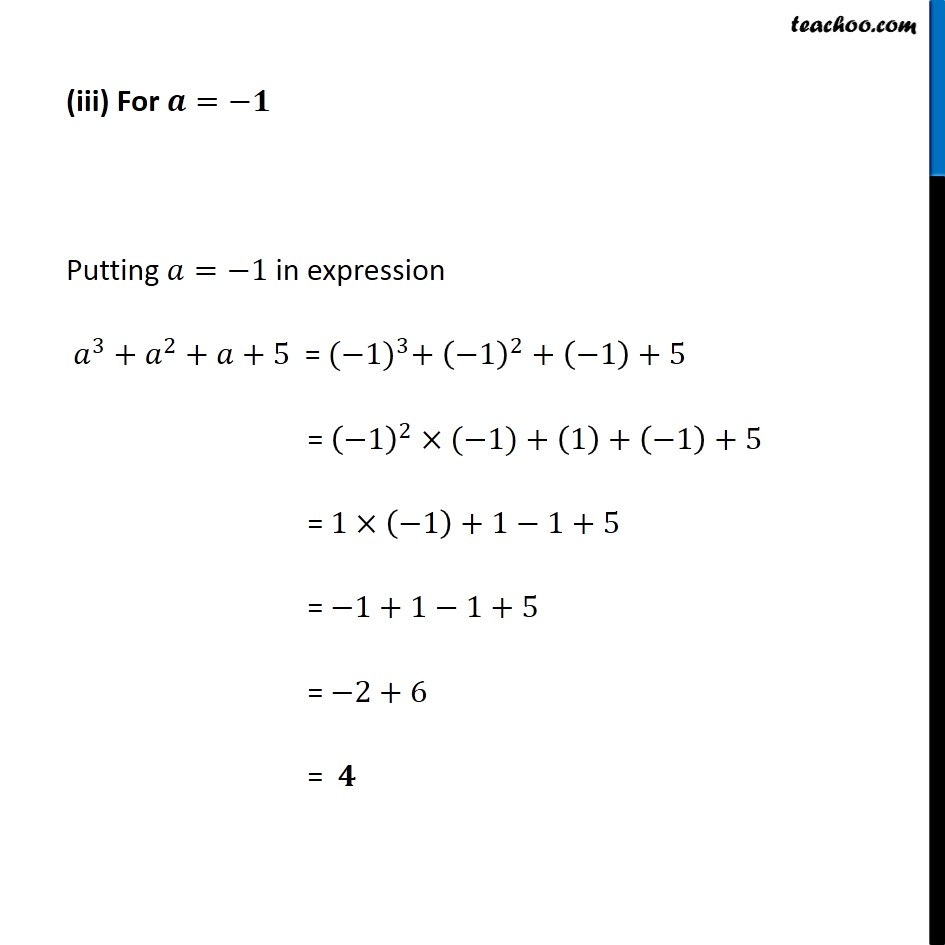Ex 8.3

Chapter 8 Class 8 Algebraic Expressions and Identities
Serial order wiseLearn in your speed, with individual attention - Teachoo Maths 1-on-1 Class

### Transcript

Ex 8.3, 4 (b) (b) Simplify a (a2 + a + 1) + 5 and find its value for (i) a = 0, (ii) a = 1 (iii) a = – 1. 𝑎 (𝑎^2+𝑎+1)+5 = (𝑎×𝑎^2 )+(𝑎×𝑎)+(𝑎×1)+5 = 𝑎^3+𝑎^2+𝑎+5 (i) For 𝒂=𝟎 Putting 𝑎=0 in expression 𝑎^3+𝑎^2+𝑎+5 = (0)^3+(0)^2+0+5 = 0+0+0+5 = 𝟓 (i) For 𝒂=𝟏 Putting 𝑎=1 in expression 𝑎^3+𝑎^2+𝑎+5 = 〖(1)〗^3+ (1)^2+1+5 = 1+1+1+5 = 𝟖 (iii) For 𝒂=−𝟏 Putting 𝑎=−1 in expression 𝑎^3+𝑎^2+𝑎+5 = 〖(−1)〗^3+ (−1)^2+(−1)+5 = (−1)^2×(−1)+(1)+(−1)+5 = 1×(−1)+1−1+5 = −1+1−1+5 = −2+6 = 𝟒